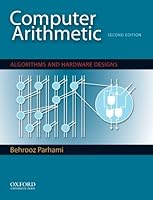# Computer Arithmetic: Algorithms and Hardware Designs, 2nd Edition## Book Description

Ideal for graduate and senior undergraduate courses in computer arithmetic and advanced digital design, Computer Arithmetic: Algorithms and Hardware Designs, Second Edition, provides a balanced, comprehensive treatment of computer arithmetic. It covers topics in arithmetic unit design and implementation that complement the architectural and algorithmic speedup techniques used in high- and parallel processing. Using a unified and consistent framework, the text begins with number representation and proceeds through basic arithmetic operations, floating-point arithmetic, and function evaluation methods. Later chapters cover broad design and implementation topics-including techniques for high-throughput, low-power, fault-tolerant, and reconfigurable arithmetic. An appendix provides a historical view of the field and speculates on its future.

An indispensable resource for instruction, professional development, and research, Computer Arithmetic: Algorithms and Hardware Designs, Second Edition, combines broad coverage of the underlying theories of computer arithmetic with numerous examples of practical designs, worked-out examples, and a large collection of meaningful problems. This second edition includes a new chapter on reconfigurable arithmetic, in order to address the fact that arithmetic functions are increasingly being implemented on field-programmable gate arrays (FPGAs) and FPGA-like configurable devices. Updated and thoroughly revised, the book offers new and expanded coverage of saturating adders and multipliers, truncated multipliers, fused multiply-add , overlapped quotient digit selection, bipartite and multipartite tables, reversible , dot notation, modular arithmetic, Montgomery modular reduction, division by constants, IEEE floating-point standard formats, and interval arithmetic.

Features:

* Divided into 28 lecture-size chapters
* Emphasizes both the underlying theories of computer arithmetic and actual hardware designs
* Carefully links computer arithmetic to other subfields of computer
* Includes 717 end-of-chapter problems ranging in complexity from simple exercises to mini-projects
* Incorporates many examples of practical designs
* Uses consistent standardized notation throughout
* Instructor's manual includes solutions to text problems
* An author-maintained website http://www.ece.ucsb.edu/~parhami/text_comp_arit.htm contains instructor resources, including complete lecture slides

PART I NUMBER REPRESENTATION
Chapter 1 Numbers and Arithmetic
Chapter 2 Representing Signed Numbers
Chapter 3 Redundant Number Systems
Chapter 4 Residue Number Systems

Chapter 5 Basic Addition and Counting
Chapter 7 Variations in Fast Adders

PART III MULTIPLICATION
Chapter 9 Basic Multiplication Schemes
Chapter 11 Tree and Array Multipliers
Chapter 12 Variations in Multipliers

PART IV DIVISION
Chapter 13 Basic Division Schemes
Chapter 15 Variations in Dividers
Chapter 16 Division by Convergence

PART V REAL ARITHMETIC
Chapter 17 Floating-Point Representations
Chapter 18 Floating-Point Operations
Chapter 19 Errors and Error Control
Chapter 20 Precise and Certifiable Arithmetic

PART VI FUNCTION EVALUATION
Chapter 21 Square-Rooting Methods
Chapter 22 The CORDIC Algorithms
Chapter 23 Variations in Function Evaluation
Chapter 24 Arithmetic by Table Lookup

PART VII IMPLEMENTATION TOPICS
Chapter 25 High-Throughput Arithmetic
Chapter 26 Low-Power Arithmetic
Chapter 27 Fault-Tolerant Arithmetic
Chapter 28 Reconfigurable Arithmetic

Appendix: Past, Present, and Future

## Book Details

• Title: Computer Arithmetic: Algorithms and Hardware Designs, 2nd Edition
• Author:
• Length: 672 pages
• Edition: 2
• Language: English
• Publisher:
• Publication Date: 2009-10-28
• ISBN-10: 0195328485
• ISBN-13: 9780195328486